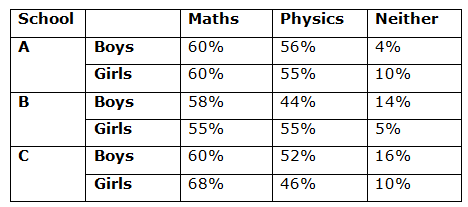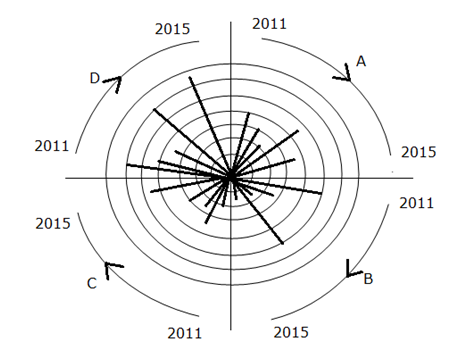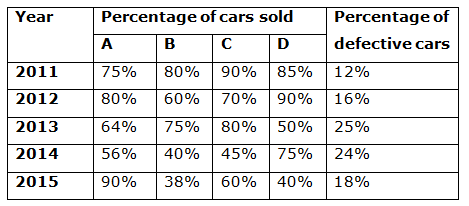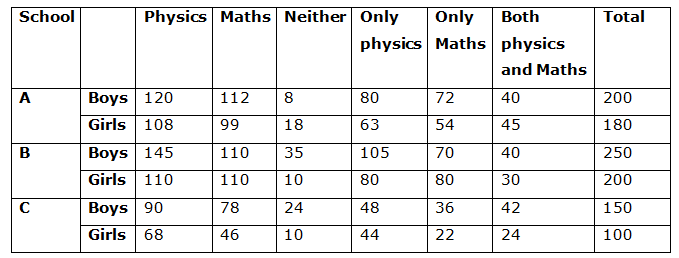# SBI PO Quantitative Aptitude Questions 2019 (Day-12) High Level New Pattern

SBI PO 2019 Notification is about to come and it is the most awaited exam among the aspirants. We all know that new pattern questions are introducing every year in the SBI PO exam. Further, the questions are getting tougher and beyond the level of the candidate’s expectations.

Our IBPS Guide is providing High-Level New Pattern Quantitative Aptitude Questions for SBI PO 2019 so the aspirants can practice it on a daily basis. These questions are framed by our skilled experts after understanding your needs thoroughly. Aspirants can practice these high-level questions daily to familiarize with the exact exam pattern. We wish that your rigorous preparation leads you to a successful target of becoming SBI PO.

[WpProQuiz 4954]

### Click Here for SBI PO Pre 2019 High-Quality Mocks Exactly on SBI Standard

Directions (1 – 5): Study the following information carefully and answer the given questions.

The following table gives the percentage distribution of number of boys and girls likes a maths or physics or neither in three schools A, B and C.• The number of boys in school A is 200 which is 11.11% more than the number of girls in the same school.
• The ratio of the number of boys to girls in school B is 5: 4. If 50 more girls joined in the class then the ratio of boys to girls becomes equal.
• The number of girls in school C is one-fourth of the total number of boys in the school A and the number of girls in the school B together and the ratio of the number of boys to girls are in the ratio 3: 2.

Note: Students like Only Maths, Only Physics, both Physics and Maths and neither Maths nor Physics.

1) Find the total number of girls like only one subject in all the three schools?

a) 334

b) 216

c) 343

d) 288

e) 224

2) In which of the following school, the difference between the number of boys and the number of girls like at least one subject, is maximum?

a) School B

b) Both School A and B

c) School C

d) Both School B and C

e) None of these

3) Quantity I: Total number of boys like only one subject in school C is what percentage more than the total number of girls like only Physics in school A?

Quantity II: The number of boys like both Physics and Maths in school A, the number of girls like both Physics and Maths in school B and the number of boys like both physics and maths in school C is what percent more/less than the number of girls like only Physics in School B?

Quantity III: The number of girls like both Physics and Maths in school C is what percentage of the number of boys like only Maths in the same school?

a) Quantity I > Quantity II ≤ Quantity III

b) Quantity I > Quantity II > Quantity III

c) Quantity I ≤ Quantity II < Quantity III

d) Quantity I > Quantity II < Quantity III

e) Quantity I ≥ Quantity II ≥ Quantity III

4) What is the difference between the total number of boys like only one subject and the total number of girls like neither Physics nor Maths?

a) 373

b) 343

c) 356

d) 442

e) 392

5) Find the ratio between the total number of students like neither Physics nor Maths and that of the total number of students like both Physics and Maths?

a) 108: 223

b) 112: 117

c) 105: 221

d) 119: 97

e) None of these

Directions (6 -10): Study the following graph carefully and answer the given questions.

The figure below shows the number of cars manufactured by four companies A, B, C and D in the year 2011 – 2015. The innermost circle represents 1000 cars; the next circle represents 2000 cars, and so on. Each represents a particular manufacture and the five lines in each quadrant correspond to the years 2011 – 2015 in clockwise order.Note: If the line is between two circles it is consider as 500 cars.

The table shows the percentage of cars sold in four companies A, B, C and D and the percentage of defective cars for all the given companies.Note: Defective cars are not consider for sales

6) Find the total number of defective cars manufactured by company B and C in the year 2012 and 2014 respectively?

Statement I: Number of defective cars manufactured in the year 2012 by company B is 50 more than company C and 150 less than company A. The number of defective cars manufactured by company D in 2012 is 18% of the total cars manufactured by company C in the same year.

Statement II: Number of defective cars manufactured by company C in the year 2014 is twice the number of defective cars manufactured by company B in the same year which is 300 less than the number of cars defective cars manufactured by company D in the same year.

a) Only I alone

b) Only II alone

c) Either I or II

d) Neither I nor II

e) Both are necessary

7) Which of the following statement is true according to the given information?

I) The difference between the number of cars sold by company B in the year 2011 and the number of cars sold by company D in the year 2013 is 2250.

II) The ratio of the total cars sold by company C in the year 2014 and 2015 together to the total cars sold by company A in the year 2013 and 2014 together is 81: 76.

III) The total number of cars manufactured by company A and B in the year 2011 is equal to the total number of cars manufactured by the same company in the year 2014.

a) Only I

b) Only III

c) Both the options (II) and (III)

d) Both the options (I) and (II)

e) All the option (I), (II) and (III)

8) In which of the following year, the number of defective cars manufactured is minimum in the given years?

a) 2012

b) 2015

c) 2013

d) 2011

e) 2014

9) Quantity I: Find the total number of cars sold by company C in the given years.

Quantity II: Find the total number of cars sold by company D in the given years.

a) Quantity I > Quantity II

b) Quantity II > Quantity I

c) Quantity II ≥ Quantity I

d) Quantity I ≥ Quantity I

e) Quantity I = Quantity II (or) No relation

10) In which of the following company has maximum increased percentage in the year 2014 from the previous year (comparing the total number of manufacturing cars)?

a) A

b) D

c) B

d) C

e) None of these

Directions (1 – 5):

School A:

Number of boys = 200

Number of boys = (100 + 11.11) % of the number of girls (Substitute the value of 100/9 instead of 11.11)

Number of girls = 200 * (900/1000) = 180

Boys:

Number of boys like Physics = (60/100)*200 = 120

Number of boys like Maths = (56/100)*200 = 112

Number of boys like neither Physics nor Maths = (4/100) * 200 = 8

Total = Only Physics + Only Maths + Neither Physics nor Maths – Both Physics and Maths

200 = 120 + 112 + 8 – Both physics and Maths

Both Physics and Maths = 240 – 200 = 40

Only Physics = 120 – 40 = 80

Only Maths = 112 – 40 = 72

Girls:

Number of girls like Physics = (60/100)*180 = 108

Number of girls like Maths = (55/100)*180 = 99

Number of girls like neither Physics nor Maths = (10/100) * 180 = 18

Total = Only Physics + Only Maths + Neither Physics nor Maths – Both Physics and Maths

180 = 108 + 99 + 18 – Both physics and Maths

Both Physics and Maths = 225 – 180 = 45

Only Physics = 108 – 45 = 63

Only Maths = 99 – 45 = 54

School B:

Number of boys and girls be 5x and 4x respectively,

According to the question,

5x / (4x+50) = 1/1

5x = 4x + 50

= > x = 50

Number of boys = 250

Number of girls = 200

Boys:

Number of boys like Physics = (58/100)*250 = 145

Number of boys like Maths = (44/100)*250 = 110

Number of boys like neither Physics nor Maths = (14/100) * 250 = 35

Total = Only Physics + Only Maths + Neither Physics nor Maths – Both Physics and Maths

250 = 145 + 110 + 35 – Both physics and Maths

Both Physics and Maths = 290 – 250 = 40

Only Physics = 145 – 40 = 105

Only Maths = 110 – 40 = 70

Girls:

Number of girls like Physics = (55/100)*200 = 110

Number of girls like Maths = (55/100)*200 = 110

Number of girls like neither Physics nor Maths = (5/100) * 200 = 10

Total = Only Physics + Only Maths + Neither Physics nor Maths – Both Physics and Maths

200 = 110 + 110 + 10 – Both physics and Maths

Both Physics and Maths = 230 – 200 = 30

Only Physics = 110 – 30 = 80

Only Maths = 110 – 30 = 80

School C:

Total number of girls in school A and B = (200 + 200) = 400

Number of girls = 400 * (1/4) = 100

Number of boys = 100 * (3/2) = 150

Boys:

Number of boys like Physics = (60/100)*150 = 90

Number of boys like Maths = (52/100)*150 = 78

Number of boys like neither Physics nor Maths = (16/100) * 150 = 24

Total = Only Physics + Only Maths + Neither Physics nor Maths – Both Physics and Maths

150 = 90 + 78 + 24 – Both physics and Maths

Both Physics and Maths = 192 – 150 = 42

Only Physics = 90 – 42 = 48

Only Maths = 78 – 42 = 36

Girls:

Number of girls like Physics = (68/100)*100 = 68

Number of girls like Maths = (46/100)*100 = 46

Number of girls like neither Physics nor Maths = (10/100) * 100 = 10

Total = Only Physics + Only Maths + Neither Physics nor Maths – Both Physics and Maths

100 = 68 + 46 + 10 – Both physics and Maths

Both Physics and Maths = 124 – 100 = 24

Only Physics = 68 – 24 = 44

Only Maths = 46 – 24 = 22Required total = (63+54+80+80+44+22) = 343

School A:

Total number of boys like at least one subject = 200 – 8 = 192

Total number of girls like at least one subject = 180 – 18 = 162

Required difference = 192 – 162 = 30

School B:

Total number of boys like at least one subject = 250 – 35 = 215

Total number of girls like at least one subject = 200 – 10 = 190

Required difference = 215 – 190 = 25

School C:

Total number of boys like at least one subject = 150 – 24 = 126

Total number of girls like at least one subject = 100 – 10 = 90

Required difference = 126 – 90 = 36

In school C has the difference between the number of boys like at least one subject and the number of girls like at least one subject is maximum.

Quantity I: Total number of boys like only one subject in school C is what percentage more than the total number of girls like only Physics in school A?

Required percentage = [(36 + 48) – 54] / 54] *100

= > (30/54)*100

= > 55.56 %

Quantity II: The number of boys like both Physics and Maths in school A, the number of girls like both Physics and Maths in school B and the number of boys like both physics and Maths in school C is what percent more/less than the number of girls like only Physics in School B?

Required percentage = {[(40+30+42) – 80]/80} * 100

= > (32/80) * 100 = 40%

Quantity III: The number of girls like both Physics and Maths in school C is what percentage of the number of boys like only Maths in the same school?

Required percentage = (24/48) * 100 = 50%

Hence, Quantity I > Quantity II < Quantity III

Total number of boys like only one subject

= (80+72+105+70+48+36)

= 411

Total number of girls like neither Physics nor Maths

= (18+10+10)

= 38

Required difference = 411 – 38 = 373

Total number of students like neither Physics nor Maths

= (8+18+35+10+24+10)

= 105

Total number of students like both Physics and Maths

= (40+45+40+30+42+24)

= 221

Required ratio = 105: 221

From statement I,

Total number of cars manufactured by all the given companies in the year 2012

= (3000+2500+3500+4000) * (16/100)

= 13000 * (16/100)

= 2080

Let us take the number of defective cars manufactured in the year 2012 by company B be x —- (1)

Number of defective cars manufactured in the year 2012 by company C

= x – 50 —- (2)

Number of defective cars manufactured in the year 2012 by company A

= x + 150 —- (3)

Number of defective cars manufactured by company D in 2012

= 3500 * (18/1000)

= 630

Total number of defective cars manufactured by company A, B and C

= 2080 – 630

A + B + C = 1450 —- (4)

Substitute the equation (1), (2) and (3) in equation (4)

(x + x – 50 + x + 150) = 1450

3x = 1450 + 50 – 150

= > 3x = 1350

= > x = 1350/3 = 450

From statement I, we can find the number of defective cars manufactured by company B in the year 2012 and there is no information about the number of defective cars manufactured by company C in the year 2014.

From statement II,

Number of defective cars manufactured by company C in the year 2014 is twice the number of defective cars manufactured by company B in the same year which is 300 less than the number of defective cars manufactured company D in the same year.

Let us take the number of defective cars manufactured by company C in the year 2014 be x

The number of defective cars manufactured by company B in the year 2014 is 2x

The number of defective cars manufactured by company D in the year 2014

= 2x + 300

From statement II, we could not find the number of defective cars manufactured by company C in the year 2014.

From I and II, both the statements are not necessary to answer the question.

From I,

Number of cars sold by company B in the year 2011

= 5000 * 80/100 = 4000

Number of cars sold by company D in the year 2013

= 3500 * 50/100 = 1750

Required difference = 4000 – 1750 = 2250

From II,

Total cars sold by company C in the year 2014 and 2015

= 3000 * 45/100 + 4500 * 60/100

= 1350 + 2700

= 4050

Total cars sold by company A in the year 2013 and 2014

= 2000 * 64/100 + 4500 * 56/100

= 1280 + 2520

= 3800

Required ratio = 4050: 3800 = 405: 380 = 81 : 76

From III,

Total number of cars manufactured by company A and B in the year 2011

= 4000 + 5000 = 9000

Total number of cars manufactured by the same company in the year 2014

= 4500 + 5000

= 9500

Total number of cars manufactured by company A and B in the year 2011 is not equal to the Total number of cars manufactured by the same company in the year 2014.

The number of defective cars manufactured in the year 2011

= (4000+5000+2000+6000)*(12/100)

= 17000 * 12/100

= 2040

The number of defective cars manufactured in the year 2012

= (3000+2500+3500+4000)*(16/100)

= 13000 * 16/100

= 2080

The number of defective cars manufactured in the year 2013

= (2000+1500+2500+3500) * 25/100

= 9500 * 25/100

= 2375

The number of defective cars manufactured in the year 2014

= (4500+5000+3000+6000)*24/100

= 18500 * 24/100

= 4440

The number of defective cars manufactured in the year 2015

= (3500+1500+4500+6500)*18/100

= 16000*18/100

= 2880

In the year 2011, the number of defective cars manufactured is minimum.

Quantity I: Find the total number of cars sold by company C in the given years.

Required total

= 2000*90/100 + 3500*70/100 + 2500*80/100 + 3000*45/100 + 4500*60/100

= 1800 + 2450 + 2000 + 1350 + 2700

= 10300

Quantity II: Find the total number of cars sold by company D in the given years.

Required total

= 6000*85/100+4000*90/100+3500*50/100+6000*75/100+6500*40/100

= 5100 + 3600 + 1750 + 4500 + 2600

= 17550

Quantity I < Quantity II

Company A:

Increased percentage = [(4500 – 2000)/2000] * 100

= (2500/2000) * 100

= 125%

Company B:

Increased percentage = [(5000 – 1500)/1500] * 100

= (3500/1500)*100

= 233.33%

Company C:

Increased percentage = [(3000 – 2500)/2500] * 100

= (500/2500) * 100

= 20%

Company D:

Increased percentage = [(6000 – 3500)/3500] * 100

= (2500/3500) * 100

= 71.42%

Company B has maximum increased percentage.

### Click Here for SBI PO Pre 2019 High-Quality Mocks Exactly on SBI Standard